Download Presentation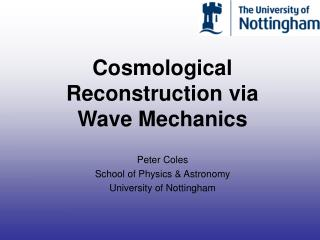Cosmological Reconstruction via Wave Mechanics

# Cosmological Reconstruction via Wave Mechanics - PowerPoint PPT Presentation

Cosmological Reconstruction via Wave Mechanics. Peter Coles School of Physics & Astronomy University of Nottingham. Cosmological Reconstruction Problems. We observe redshifts and (sometimes) estimated distances in the evolved local Universe for some galaxiesI am the owner, or an agent authorized to act on behalf of the owner, of the copyrighted work described.
Download Presentation## Cosmological Reconstruction via Wave Mechanics

An Image/Link below is provided (as is) to download presentation

Download Policy: Content on the Website is provided to you AS IS for your information and personal use and may not be sold / licensed / shared on other websites without getting consent from its author.While downloading, if for some reason you are not able to download a presentation, the publisher may have deleted the file from their server.

- - - - - - - - - - - - - - - - - - - - - - - - - - E N D - - - - - - - - - - - - - - - - - - - - - - - - - -
Presentation Transcript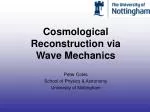Cosmological Reconstruction via Wave Mechanics

Peter Coles

School of Physics & Astronomy

University of Nottingham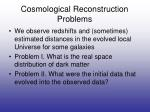Cosmological Reconstruction Problems
• We observe redshifts and (sometimes) estimated distances in the evolved local Universe for some galaxies
• Problem I. What is the real space distribution of dark matter
• Problem II. What were the initial data that evolved into the observed data?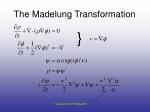Growing mode

Decaying mode

The fluid approach
• Cold Dark Matter evolves according to a Vlasov equation coupled to a Poisson equation for the gravitational potential
• The Vlasov-Poisson system is hard, so treat collisionless CDM as a fluid…
• Linear perturbation theory gives an equation for the density contrast
• In a spatially flat CDM-dominated universe

where:

• Comoving velocity associated with the growing mode is irrotational: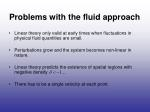Problems with the fluid approach
• Linear theory only valid at early times when fluctuations in physical fluid quantities are small.
• Perturbations grow and the system becomes non-linear in nature.
• Linear theory predicts the existence of spatial regions with negative density …
• There has to be a single velocity at each point.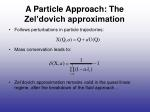A Particle Approach: The Zel’dovich approximation
• Follows perturbations in particle trajectories:
• Mass conservation leads to:
• Zel’dovich approximation remains valid in the quasi-linear regime, after the breakdown of the fluid approach…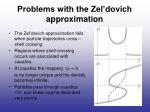Problems with the Zel’dovich approximation
• The Zel’dovich approximation fails when particle trajectories cross – shell crossing.
• Regions where shell-crossing occurs are associated with caustics.
• At caustics the mapping

is no longer unique and the density becomes infinite.

• Particles pass through caustics non-linear regime described very poorly.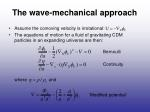Bernoulli

Continuity

‘Modified potential’

The wave-mechanical approach

• Assume the comoving velocity is irrotational:
• The equations of motion for a fluid of gravitating CDM particles in an expanding universe are then:

where andThe wave-mechanical approach

• Apply the Madelung transformation

to the fluid equations.

• Obtain the Schrodinger equation:
• the quantum pressure term
• the De Broglie wavelength
• It’s possible to add polytropic gas pressure too…using the Gross-Pitaevskii equationThe ‘free-particle’ Schrodinger equation
• In a spatially flat CDM-dominated universe, the ‘potential’

in the linear regime; see Coles & Spencer (2003, MNRAS, 342, 176)

• Neglecting quantum pressure, the Schrodinger equation to be solved is then the ‘free-particle’ equation:
• Can be solved exactly: quantum-mechanical analogue of the Zel’dovich approximation.Why bother?
• An example: why is the density field so lognormal?
• Very easy to see using this representation: Coles (2002, MNRAS, 329, 37); see also Szapudi & Kaiser (2003, ApJ, 583, L1).Gravitational collapse in one dimension
• Assume a sinusoidal initial density profile in 1D:

where is the comoving period of the perturbation.

• Free parameters are:

1. The amplitude of the initial density fluctuation.

2. The dimensionless number

• Quantum pressure
• DeBroglie wavelengthGravitational collapse in one dimension

Evolution of a periodic 1D self-gravitating system withRelation to Classical Fluids
• Write
• Then, ignoring quantum pressure and having =1
• and define a velocity

All trajectories on which A20 define a velocity field; the classical trajectories are streamlines of a probability flowStreamlines and Solutions
• Suppose such a streamline is a(t).
• Any point (x,t) can be written
• Then
• Ignoring higher order terms
• So

Quantum Oscillation

Classical PhaseThe Trouble with 
• The classical limit has 0…
• BUT the “weight” oscillates wildly as this limit is approached.
• For a finite computation, need a finite value of 
• Also, system becomes “non-perturbative”
• Quantum Turbulence!
• Note  is dimensionally a viscosity; c.f. Burgers equationZel’dovich-Bernoulli

Wave Mechanics and the Zel’dovich-Bernoulli method
• In Eulerian space the Zel’dovich approximation becomes:
• One method of doing reconstruction..
• The Zel’dovich-Bernoulli equation can be replaced by the ‘free-particle’ Schrodinger equation..
• ..detailed tests of this are in progress (Short & Coles, in prep).Cosmic reconstruction
• Gravity is invariant under time-reversal!
• Unitarity means density is always well-behaved.
• The reconstruction question:
• Non-linear gravitational evolution is a major obstacle to reconstruction.
• Non-linear multi-stream regions prevent unique reconstruction.
• At scales above a few Mpc, multi-streaming is insignificant

smoothing necessary.

Given the large-scale structure observable today, can we reverse the effects of gravity and recover information about the primordial universe?Further reconstruction
• This is a very limited application of this idea.
• Still one fluid velocity at each spatial position.
• To go further we need to represent the distribution function and solve the Vlasov equation.
• This needs a more sophisticated representation, e.g. coherent state (Wigner, Husimi)The wave-mechanical approach
• For a collisionless medium, shell-crossing leads to the generation of vorticity velocity flow no longer irrotational
• Possible to construct more sophisticated representations of the wavefunction that allow for multi-streaming (Widrow & Kaiser 1993).

Phase-space evolution of a 1D self-gravitating system with ,Fuzzy Dark Matter
• It is even possible that Dark Matter is made of a very light particle with an effective compton wavelength comparable to a galactic scale.
• Dark matter then forms a kind of condensate, but quantum behaviour prevents cuspy cores.
• The quantum of vorticity is also huge…..and another thing
• Non-linear Schrödinger (Gross-Pitaevskii) equation

In fluid description, this gives pressure forces arising from a polytropic gas.Summary
• The wave-mechanical approach can overcome some of the main difficulties associated with the fluid approach and the Zel’dovich approximation.
• More sophisticated representations of the wavefunction can be used to allow for multi-streaming.
• The quantum pressure term is crucial in determining how well the wave-mechanical approach performs.
• The `free-particle’ Schrodinger equation can be applied to the problem of reconstruction.